1
57
views
51b
Problem

Problem 51b

Chapter 1: Functions and ModelsTextbook ExpertVerified Tutor
14 Oct 2021

Given information

Given logarithmic equation is,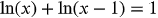Step-by-step explanation

Step 1.

On simplification, we get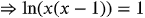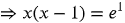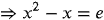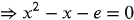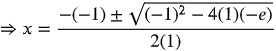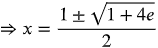But the given equation is not defined when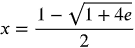.

Therefore the solution is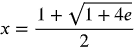.